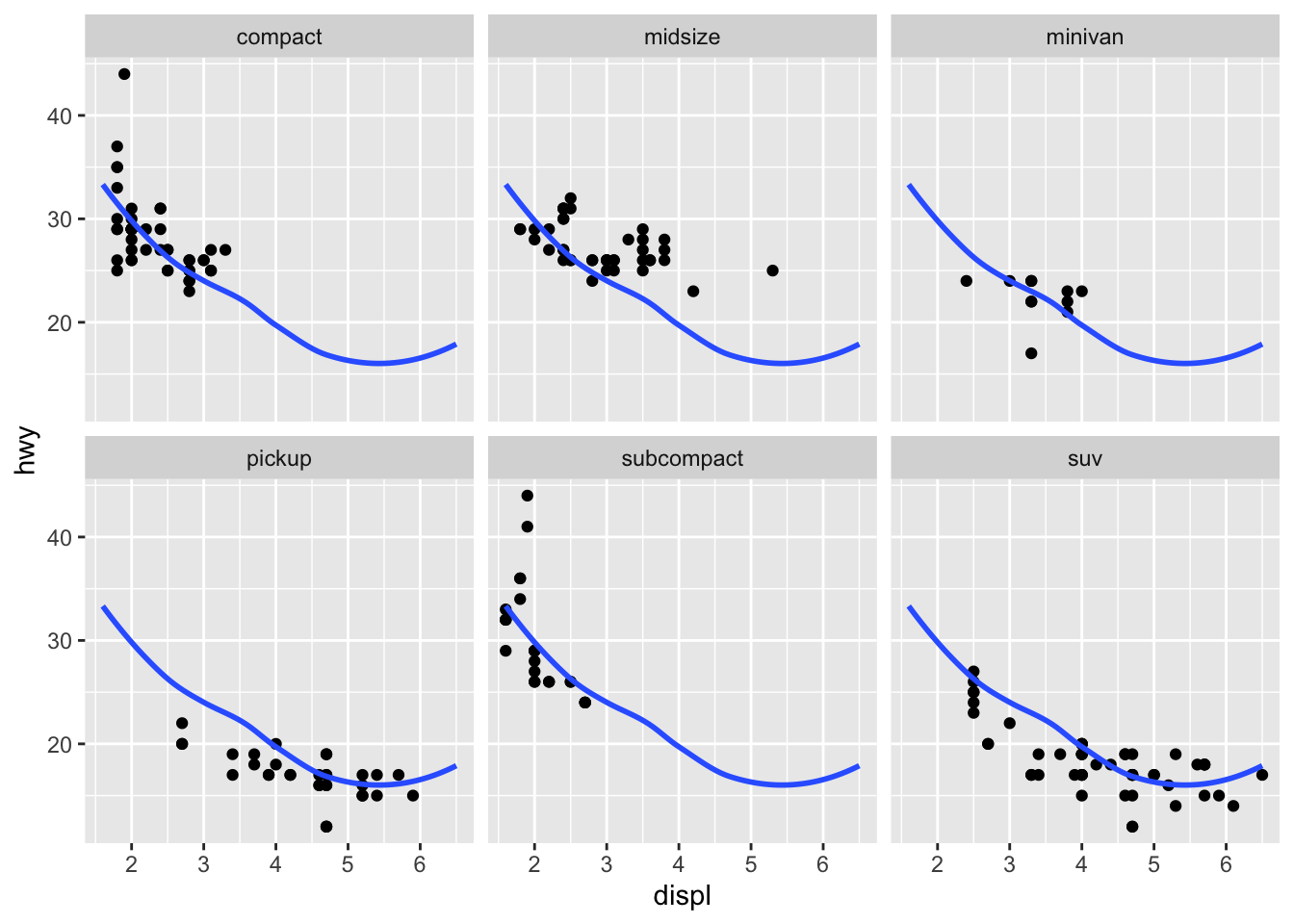# 17 Faceting

## 17.1 Exercises

1.

``````# faceting by cut and grouping by carat.
diamonds %>%
ggplot(aes(price)) +
geom_histogram(aes(color = carat)) +
facet_wrap(~cut, scales = "free_y")
#> `stat_bin()` using `bins = 30`. Pick better value with
#> `binwidth`.``````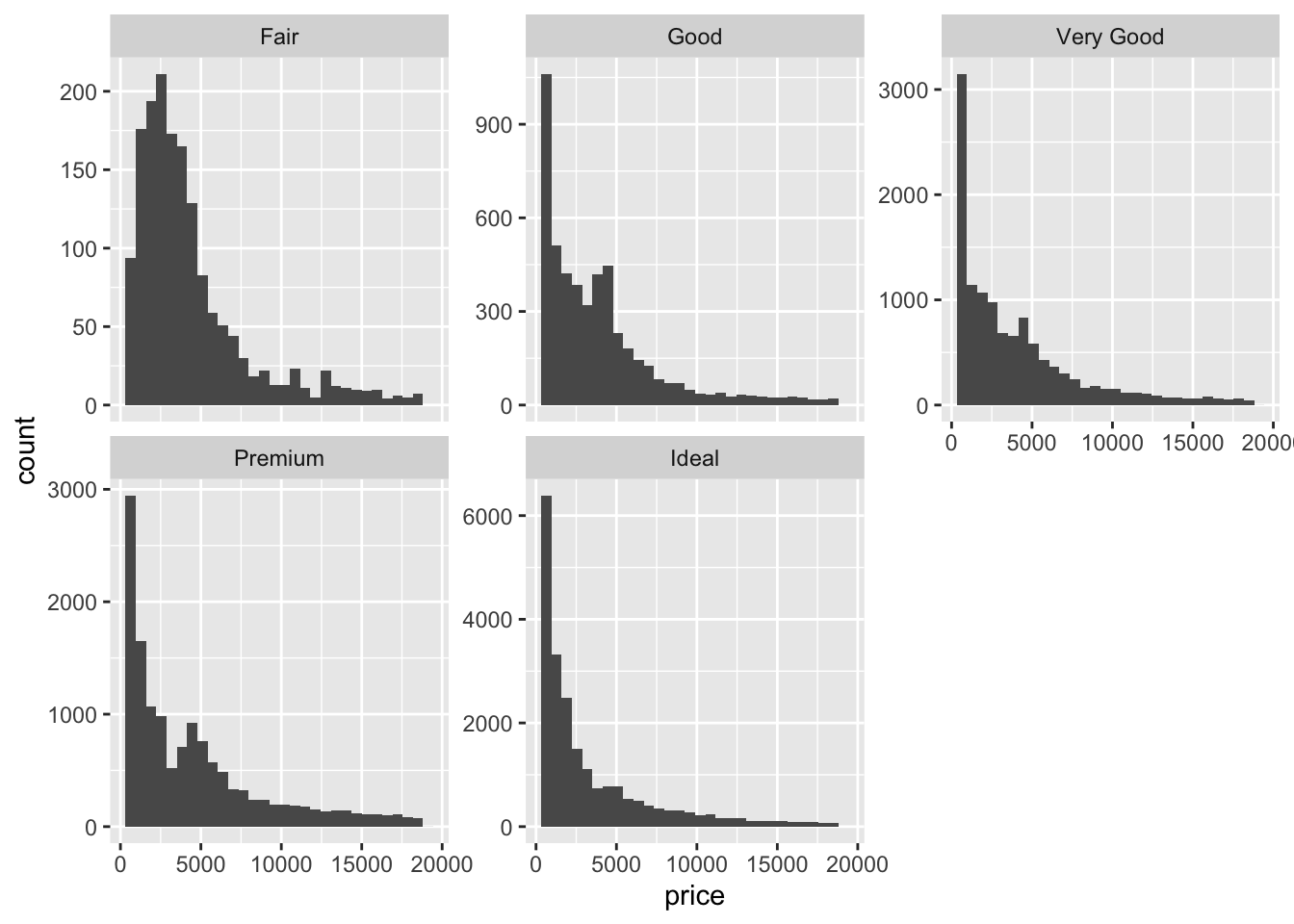``````
# faceting by carat and grouping by cut.
diamonds %>%
ggplot(aes(price)) +
geom_histogram(aes(color = cut)) +
facet_wrap(~carat, scales = "free_y")
#> `stat_bin()` using `bins = 30`. Pick better value with
#> `binwidth`.``````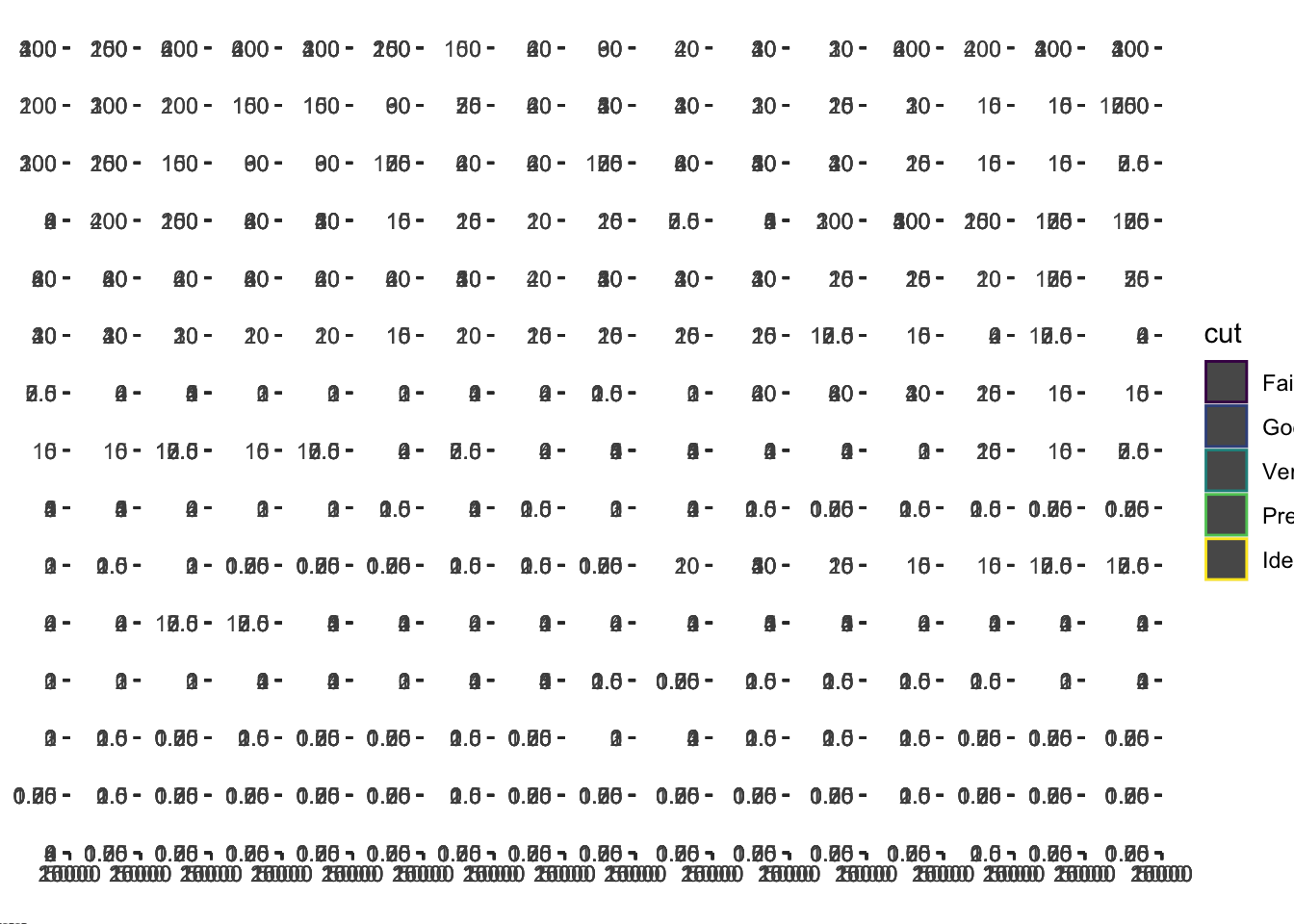• It makes more sense to facet by cut because its a discrete variable. Faceting by carat, a continuous variable, makes too many facets and renders the plot unreadable!

2.

``````diamonds %>%
ggplot(aes(carat, price)) +
geom_point(aes(color = color))``````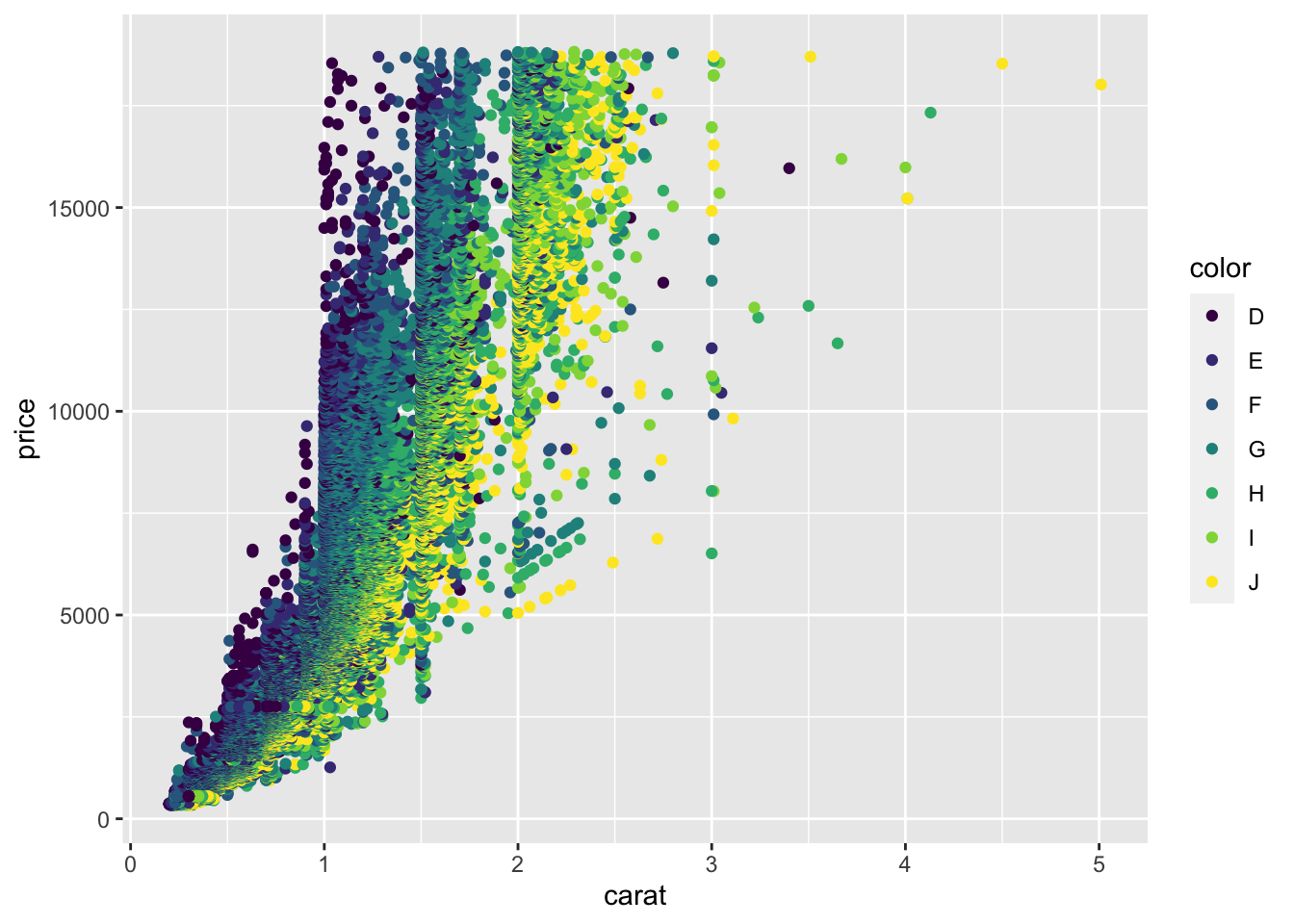``````diamonds %>%
ggplot(aes(carat, price)) +
geom_point(aes(color = color)) +
facet_wrap(~color)``````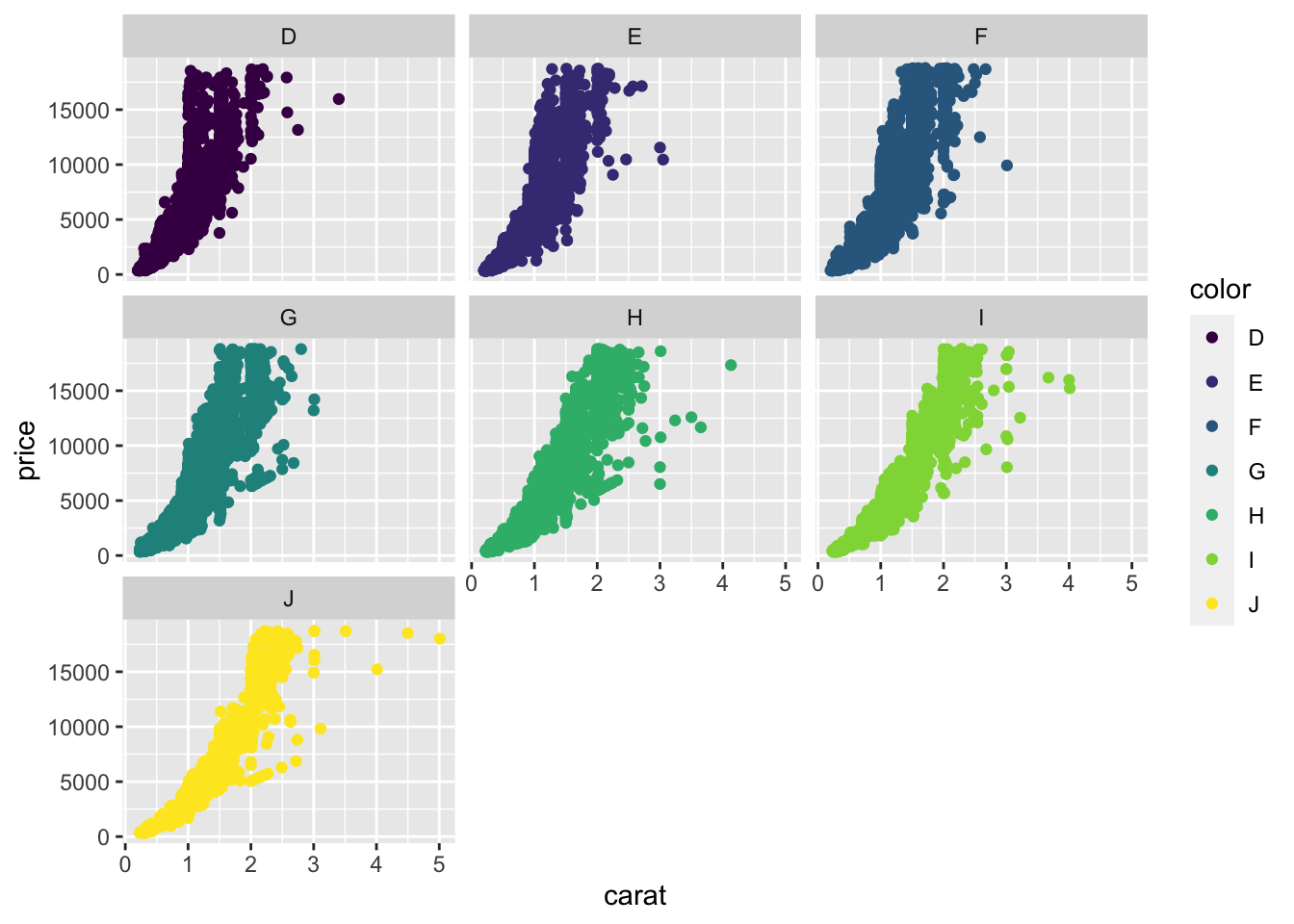• I think its better to use grouping to compare the different colors. The panels all have the same shape, so it’s hard to compare the groups across facets. If I use faceting, I’d add that the plot is facetted by diamond colour, from D (best) to J (worst).

3. I think `facet_wrap()` is more useful than `facet_grid()` because the former function is useful if you have a single variable with many levels and want to arrange the plots in a more space efficient manner. In data analysis, its extremely common to have a single variable with many levels that the analyst wants to arrange the for easy comparison. Although `facet_grid()` works on single variables, `facet_wrap()` involves less typing when you have a single variable.

4.

``````mpg2 <- subset(mpg, cyl != 5 & drv %in% c("4", "f") & class != "2seater")

mpg2 %>%
ggplot(aes(displ, hwy)) +
geom_point() +
geom_smooth(data = mpg2 %>% select(-class),
se = FALSE,
method = "loess") +

facet_wrap(~class)
#> `geom_smooth()` using formula 'y ~ x'``````